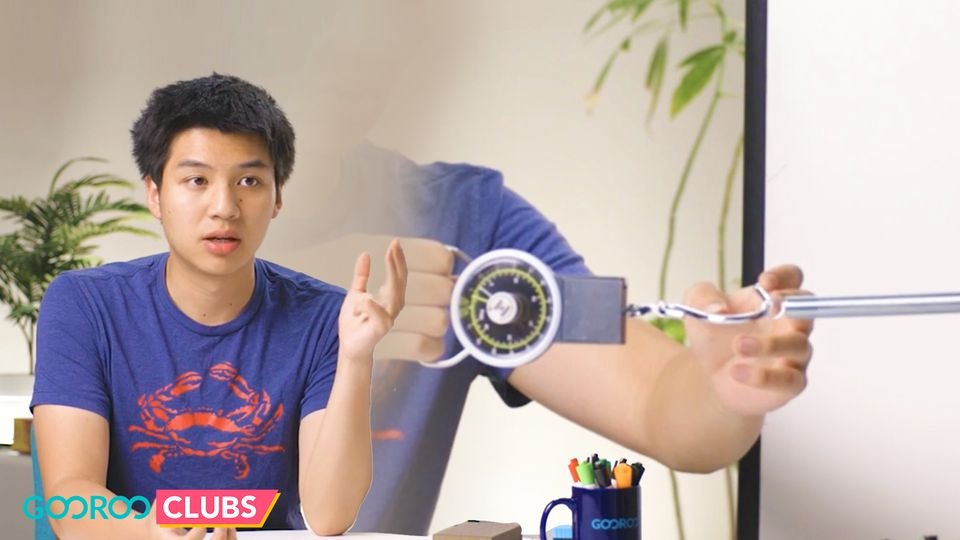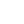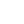Preview the Course
8m

# Forces and Interactions: Friction

by Max S. | Science3 Lessons 8:33
1.1. Normal Force 3:18
2.2. Introduction to friction 2:53
3.3. Static Friction vs Kinetic Friction 2:22

8m

What prevents a parked car from rolling down a hill? How do objects relate to each other in space? Learn about the integral elements of normal force, and get a primer on friction forces. Get to know the three types of friction forces and how they differ from each other.

Magnets, electricity, force, and friction - master the crucial concepts of Forces and Interactions!

### Project

Basic math and geometry

### Course overview

1

Normal Force

Students will understand how normal force counteracts gravity and propels objects down slopes.

2

Introduction to friction

Students will understand how friction acts as a force and how it is determined by weight and surface.

3

Static Friction vs Kinetic Friction

Students will learn to identify the difference between static and kinetic friction.

See more#### Quiz

Assess your learning with a quiz.

Tags:

• Friction
• Friction Forces
• Gravity
• Physics
• Science

## Recommended Materials

• Blocks
• Sandpaper
• Sloped surfaces
• Spring scale

## Instructor

Max is a physics enthusiast and rising senior at Rutgers University. Academically, he is most intrigued by the intuitive and approachable nature of forces and mechanics. Outside of physics, Max likes to stay active and follow sports.

See full profile